# boolean algebra

## Boolean algebraic identities

In mathematics, an identity is a statement true for all possible values of its variable or variables. The algebraic identity of x + 0 = x tells us that anything (x) added to zero equals the original “anything,” no matter what value that “anything” (x) may be. Like ordinary algebra, Boolean algebra has its own unique identities based on the bivalent states of Boolean variables.

The first Boolean identity is that the sum of anything and zero is the same as the original “anything.” This identity is no different from its eal-number algebraic equivalent: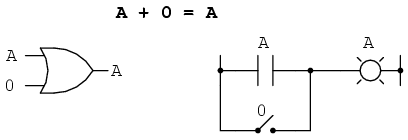No matter what the value of A, the output will always be the same: when A=1, the output will also be 1; when A=0, the output will also be 0.

The next identity is most definitely different from any seen in normal algebra. Here we discover that the sum of anything and one is one: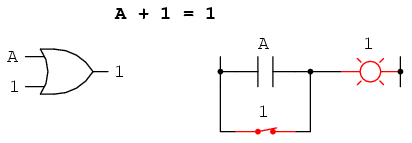No matter what the value of A, the sum of A and 1 will always be 1. In a sense, the “1” signal overrides the effect of A on the logic circuit, leaving the output fixed at a logic level of 1.

Next, we examine the effect of adding A and A together, which is the same as connecting both inputs of an OR gate to each other and activating them with the same signal: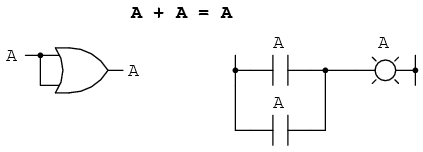In real-number algebra, the sum of two identical variables is twice the original variable’s value (x + x = 2x), but remember that there is no concept of “2” in the world of Boolean math, only 1 and 0, so we cannot say that A + A = 2A. Thus, when we add a Boolean quantity to itself, the sum is equal to the original quantity: 0 + 0 = 0, and 1 + 1 = 1.

Introducing the uniquely Boolean concept of complementation into an additive identity, we find an interesting effect. Since there must be one “1” value between any variable and its complement, and since the sum of any Boolean quantity and 1 is 1, the sum of a variable and its complement must be 1: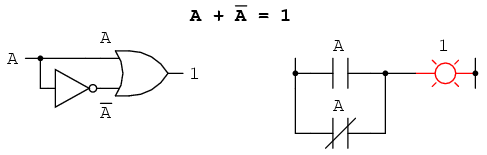Just as there are four Boolean additive identities (A+0, A+1, A+A, and A+A’), so there are also four multiplicative identities: Ax0, Ax1, AxA, and AxA’. Of these, the first two are no different from their equivalent expressions in regular algebra: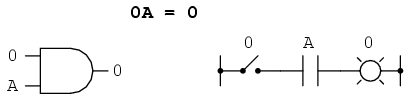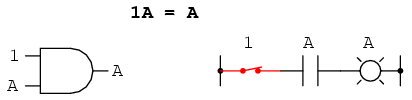The third multiplicative identity expresses the result of a Boolean quantity multiplied by itself. In normal algebra, the product of a variable and itself is the square of that variable (3 x 3 = 32 = 9). However, the concept of “square” implies a quantity of 2, which has no meaning in Boolean algebra, so we cannot say that A x A = A2. Instead, we find that the product of a Boolean quantity and itself is the original quantity, since 0 x 0 = 0 and 1 x 1 = 1: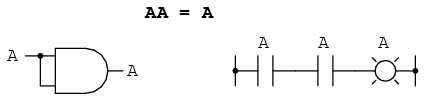The fourth multiplicative identity has no equivalent in regular algebra because it uses the complement of a variable, a concept unique to Boolean mathematics. Since there must be one “0” value between any variable and its complement, and since the product of any Boolean quantity and 0 is 0, the product of a variable and its complement must be 0: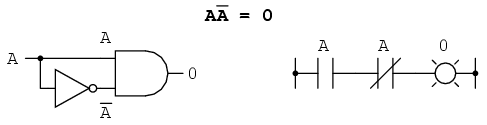To summarize, then, we have four basic Boolean identities for addition and four for multiplication: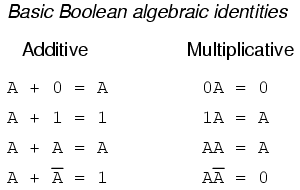Another identity having to do with complementation is that of the double complement: a variable inverted twice. Complementing a variable twice (or any even number of times) results in the original Boolean value. This is analogous to negating (multiplying by -1) in real-number algebra: an even number of negations cancel to leave the original value: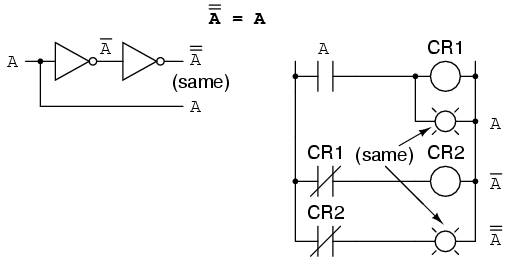## Boolean algebraic properties

Another type of mathematical identity, called a “property” or a “law,” describes how differing variables relate to each other in a system of numbers. One of these properties is known as the commutative property, and it applies equally to addition and multiplication. In essence, the commutative property tells us we can reverse the order of variables that are either added together or multiplied together without changing the truth of the expression: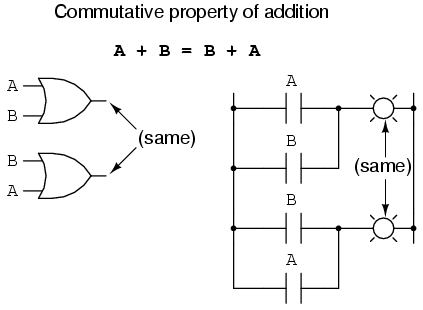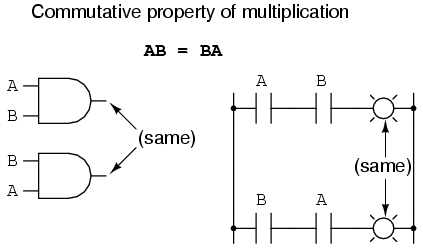Along with the commutative properties of addition and multiplication, we have the associative proprty, again applying equally well to addition and multiplication. This property tells us we can associate groups of added or multiplied variables together with parentheses without altering the truth of the equations.Lastly, we have the distributive property, illustrating how to expand a Boolean expression formed by the product of a sum, and in reverse shows us how terms may be factored out of Boolean sums-of-products:To summarize, here are the three basic properties: commutative, associative, and distributive.## Boolean rules for simplification

Boolean algebra finds its most practical use in the simplification of logic circuits. If we translate a logic circuit’s function into symbolic (Boolean) form, and apply certain algebraic rules to the resulting equation to reduce the number of terms and/or arithmetic operations, the simplified equation may be translated back into circuit form for a logic circuit performing the same function with fewer components. If equivalent function may be achieved with fewer components, the result will be increased reliability and decreased cost of manufacture.

To this end, there are several rules of Boolean algebra presented in this section for use in reducing expressions to their simplest forms. The identities and properties already reviewed in this chapter are very useful in Boolean simplification, and for the most part bear similarity to many identities and properties of “normal” algebra. However, the rules shown in this section are all unique to Boolean mathematics.This rule may be proven symbolically by factoring an “A” out of the two terms, then applying the rules of A + 1 = 1 and 1A = A to achieve the final result:Please note how the rule A + 1 = 1 was used to reduce the (B + 1) term to 1. When a rule like “A + 1 = 1” is expressed using the letter “A”, it doesn’t mean it only applies to expressions containing “A”. What the “A” stands for in a rule like A + 1 = 1 is any Boolean variable or collection of variables. This is perhaps the most difficult concept for new students to master in Boolean simplification: applying standardized identities, properties, and rules to expressions not in standard form.

For instance, the Boolean expression ABC + 1 also reduces to 1 by means of the “A + 1 = 1” identity. In this case, we recognize that the “A” term in the identity’s standard form can represent the entire “ABC” term in the original expression.

The next rule looks similar to the first on shown in this section, but is actually quite different and requires a more clever proof: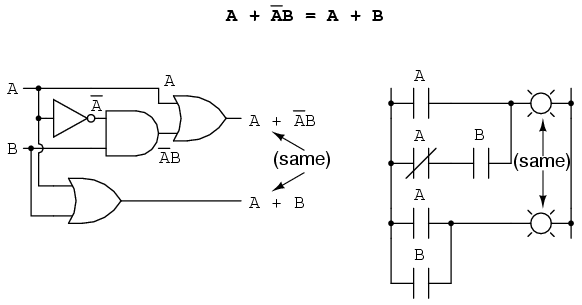Note how the last rule (A + AB = A) is used to “un-simplify” the first “A” term in the expression, changing the “A” into an “A + AB”. While this may seem like a backward step, it certainly helped to reduce the expression to something simpler! Sometimes in mathematics we must take “backward” steps to achieve the most elegant solution. Knowing when to take such a step and when not to is part of the art-form of algebra, just as a victory in a game of chess almost always requires calculated sacrifices.

Another rule involves the simplification of a product-of-sums expression: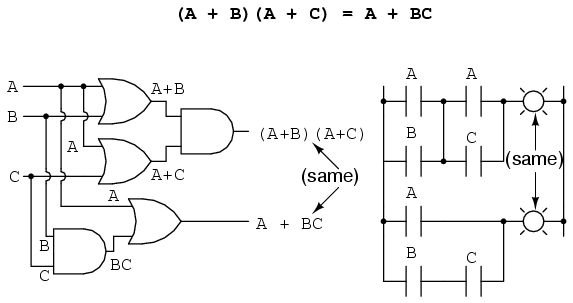And, the corresponding proof:To summarize, here are the three new rules of Boolean simplification expounded in this section:## Circuit simplification examples

Let’s begin with a semiconductor gate circuit in need of simplification. The “A,” “B,” and “C” input signals are assumed to be provided from switches, sensors, or perhaps other gate cicuits. Where these signals originate is of no concern in the task of gate reduction.Our first step in simplification must be to write a Boolean expression for this circuit. This task is easily performed step by step if we start by writing sub-expressions at the output of each gate, corresponding to the respective input signals for each gate. Remember that OR gates are equivalent to Boolean addition, while AND gates are equivalent to Boolean multiplication. For example, I’ll write sub-expressions at the outputs of the first three gates:. . . then another sub-expression for the next gate:Finally, the output (“Q”) is seen to be equal to the expression AB + BC(B + C):Now that we have a Boolean expression to work with, we need to apply the rules of Boolean algebra to reduce the expression to its simplest form (simplest defined as requiring the fewest gates to implement):The final expression, B(A + C), is much simpler than the original, yet performs the same function. If you would like to verify this, you may generate a truth table for both expressions and determine Q’s status (the circuits’ output) for all eight logic-state combinations of A, B, and C, for both circuits. The two truth tables should be identical.

Now, we must generate a schematic diagram from this Boolean expression. To do this, evaluate the expression, following proper mathematical order of operations (multiplication before addition, operations inside parentheses before anything else), and draw gates for each step. Remember again that OR gates are equivalent to Boolean addition, while AND gates are equivalent to Boolean multiplication. In this case, we would begin with the sub-expression “A + C”, which is an OR gate:The next step in evaluating the expression “B(A + C)” is to multiply (AND gate) the signal B by the output of the previous gate (A + C):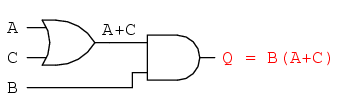Obviously, this circuit is much simpler than the original, having only two logic gates instead of five. Such component reduction results in higher operating speed (less delay time from input signal transition to output signal transition), less power consumption, less cost, and greater reliability.

Electromechanical relay circuits, typically being slower, consuming more electrical power to operate, costing more, and having a shorter average life than their semiconductor counterparts, benefit dramatically from Boolean simplification. Let’s consider an example circuit: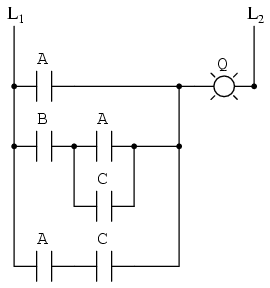As before, our first step in reducing this circuit to its simplest form must be to develop a Boolean expression from the schematic. The easiest way I’ve found to do this is to follow the same steps I’d normally follow to reduce a series-parallel resistor network to a single, total resistance. For example, examine the following resistor network with its resistors arranged in the same connection pattern as the relay contacts in the former circuit, and corresponding total resistance formula:Remember that parallel contacts are equivalent to Boolean addition, while series contacts are equivalent to Boolean multiplication. Write a Boolean expression for this relay contact circuit, following the same order of precedence that you would follow in reducing a series-parallel resistor network to a total resistance. It may be helpful to write a Boolean sub-expression to the left of each ladder “rung,” to help organize your expression-writing:Now that we have a Boolean expression to work with, we need to apply the rules of Boolean algebra to reduce the expression to its simplest form (simplest defined as requiring the fewest relay contacts to implement):The more mathematically inclined should be able to see that the two steps employing the rule “A + AB = A” may be combined into a single step, the rule being expandable to: “A + AB + AC + AD + . . . = A”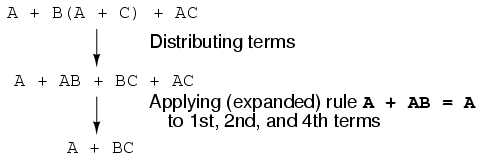As you can see, the reduced circuit is much simpler than the original, yet performs the same logical function:• REVIEW:
• To convert a gate circuit to a Boolean expression, label each gate output with a Boolean sub-expression corresponding to the gates’ input signals, until a final expression is reached at the last gate.
• To convert a Boolean expression to a gate circuit, evaluate the expression using standard order of operations: multiplication before addition, and operations within parentheses before anything else.
• To convert a ladder logic circuit to a Boolean expression, label each rung with a Boolean sub-expression corresponding to the contacts’ input signals, until a final expression is reached at the last coil or light. To determine proper order of evaluation, treat the contacts as though they were resistors, and as if you were determining total resistance of the series-parallel network formed by them. In other words, look for contacts that are either directly in series or directly in parallel with each other first, then “collapse” them into equivalent Boolean sub-expressions before proceeding to other contacts.
• To convert a Boolean expression to a ladder logic circuit, evaluate the expression using standard order of operations: multiplication before addition, and operations within parentheses before anything else.

## The Exclusive-OR function

One element conspicuously missing from the set of Boolean operations is that of Exclusive-OR. Whereas the OR function is equivalent to Boolean addition, the AND function to Boolean multiplication, and the NOT function (inverter) to Boolean complementation, there is no direct Boolean equivalent for Exclusive-OR. This hasn’t stopped people from developing a symbol to represent it, though: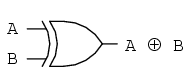This symbol is seldom used in Boolean expressions because the identities, laws, and rules of simplification involving addition, multiplication, and complementation do not apply to it. However, there is a way to represent the Exclusive-OR function in terms of OR and AND, as has been shown in previous chapters: AB’ + A’BAs a Boolean equivalency, this rule may be helpful in simplifying some Boolean expressions. Any expression following the AB’ + A’B form (two AND gates and an OR gate) may be replaced by a single Exclusive-OR gate.

Related

COMMENT songs

1. Anonymous

Is
A’ + AB = A + B?